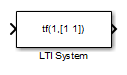# LTI System

Use linear time invariant system model object in Simulink

• Library:
• Control System Toolbox

•## Description

The LTI System block imports linear system model objects into the Simulink® environment. You specify the LTI model to import in the LTI system variable parameter. You can import any type of proper linear time-invariant dynamic system model. If the imported system is a state-space (`ss`) model, you can specify initial state values in the Initial states parameter.

## Ports

### Input

expand all

For a single-input LTI system, the input signal is a scalar. For multiple-input systems, combine the system inputs into a vector signal, using blocks such as:

### Output

expand all

For a single-output LTI system, the output signal is a scalar. For multiple-output systems, the output signal is a vector. To split system outputs into scalar signals, use blocks such as:

## Parameters

expand all

Specify the linear system for the block as a MATLAB® expression or a variable in the MATLAB workspace, the model workspace, or a data dictionary. The model can be SISO or MIMO.

Most linear time-invariant dynamic system models are supported, except:

• Frequency-response data models, such as `frd` and `genfrd` models.

• Nonlinear identified models, such as `idnlarx`.

• Models with unmodeled dynamics, such as `udyn`.

The specified model must be proper (see `isproper`).

The model can be either continuous time or discrete time. When the LTI system block is in a Simulink model with synchronous state control (see the State Control (HDL Coder) block), you must specify a discrete-time model.

Simulink converts the model to its state-space equivalent prior to initializing the simulation.

If the linear system is in state-space form, specify the initial state values as a vector with as many entries as the system has states. If you specify a scalar value, the block applies that value to each state in the system. The default value, `[]`, initializes all states to zero.

The concept of initial state is not well-defined for linear systems that are not in state-space form, such as transfer functions or zero-pole-gain models. For such models, the initial state depends on the choice of state coordinates used by the realization algorithm. As a result, the block ignores this parameter for such models.

## Version History

Introduced before R2006a